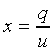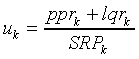Standard practice will generally be to quote prepayment in terms of the separate annualized rates PPR and LQR. However, at times it is also useful to quote prepayment in terms of an annualized total prepayment rate, denoted UPP, and the fraction of prepayments that are due to liquidations, denoted x.

As can be seen from equation , total monthly prepayments, u, are related to partial prepayments p and liquidations q in the following manner:

(1-u)=(1-q)·(1-p)

or

u=1-(1-q)·(1-p) 

The fraction of total prepayments that is due to liquidations is calculated as:Given a constant rate, u, the annualized total unscheduled principal prepayment rate (UPP) is calculated:

UPP=[1-(1-u)12]·100 

Monthly rates, uk, can be calculated from historical cash flows:Appendix 3 provides additional discussion on the relationship between UPP and the separate rates PPR and LQR.

Date Published:: March 31, 2018